# Class 12 Maths NCERT Solutions for Chapter 4 Determinants Miscellaneous Exercise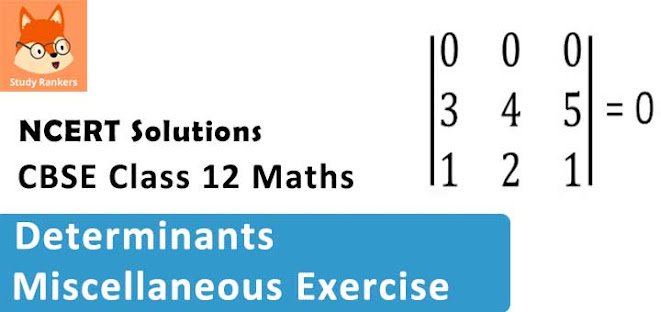### Determinants Miscellaneous Exercise Solutions

1. Prove that the determinant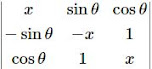is independent of θ is independent of θ.

Solution

Δ =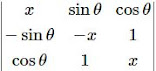= x(–x2 – 1) – sinθ(– x sinθ – cos θ) + cos θ(– sinθ + x cosθ)
= –x3 – x + xsin2 θ + sinθ cosθ – sinθ cosθ + x cos2θ
= –x3 – x + x(sin2θ + cos2θ)
= –x3 – x + x
= –x3 (Independent of θ)
Hence, Δ is independent of θ.

2. Without expanding the determinant, prove that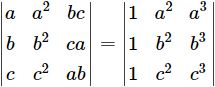Solution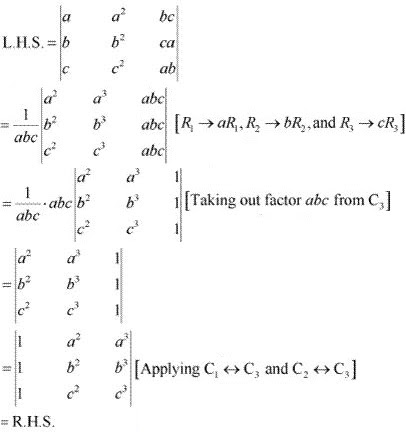Hence, the given result is proved.

3. Evaluate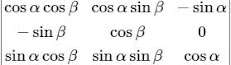Solution  Δ =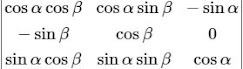Expanding along C3 , we have :
∆ = − sin α(−sin α sin2β – cos2 β sinα) + cos α(cos α cos2 β + cos α sin2 β)
= sin2 α(sin2 β + cos2 β) + cos2 α(cos2 β + sin2 β)
= sin2 α(1) + cos2 α(1)
= 1

4. If a, b and c are real numbers, and triangle  =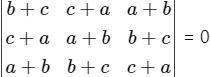Show that either a + b + c = 0 or a = b = c.
Solution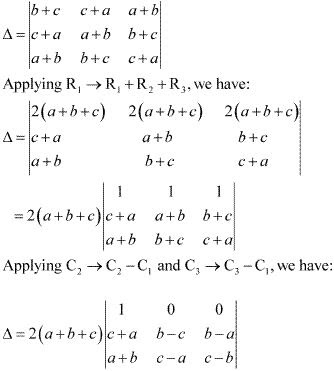Expanding along R1 , we have :
Δ = 2 (a + b + c)(1)[(b - c)(c - b) - (b - a)(c - a)]
= 2(a + b + c)[-b2 - c2 + 2bc - bc + ba + ac - a2 ]
= 2(a + b + c)[ab + bc +  ca - a2  - b2  - c2 ]
It is given that Δ = 0.
(a + b + c)[ab + bc + ca - a2 - b2 - c2] = 0
⇒ Either a + b + c = 0, or ab + bc + ca - a2 - b2 - c2 = 0 .
Now,
ab + bc + ca - a2 - b2 - c2 = 0
⇒ -2ab - 2bc - 2ca + 2a2 + 2b2 + 2c2  = 0
⇒ (a - b)2 + (b - c)2 + (c - a)2 = 0
⇒ (a - b)2 = (b - c)2 = (c - a)2  = 0 [(a-b)2, (b-c)2, (c-a)2 are non-negative]
⇒ (a - b) = (b - c) = (c - a) = 0
⇒ a = b = c
Hence, if Δ = 0 then either a + b + c = 0 or a = b = c.

5. Solve the equations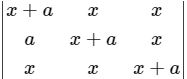= 0, a ≠ 0
Solution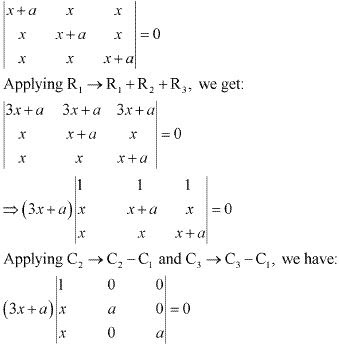Expanding along R1 , we have :
(3x + a)[1 × a2 ]= 0
⇒ a2 (3x + a) = 0
But a ≠ 0.
Therefore, we have :
3x + a = 0
⇒ x = -a/3

6. Prove that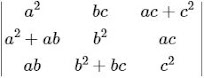= 4a2 b2 c2
Solution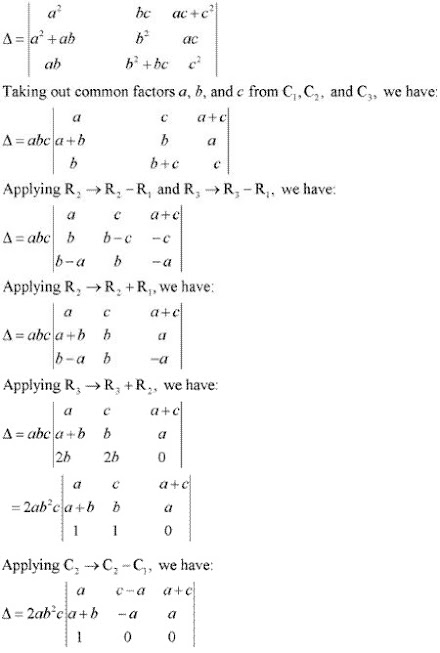Expanding along R3 , we have :
Δ = 2ab2 c[a(c - a) + a(a + c)]
= 2ab2 c[ac - a2 + a2 + ac]
= 2ab2 c(2ac)
= 4a2 b2 c2
Hence, the given result is proved.

7. If A ^(-1) =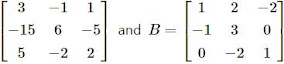find (AB)-1 .
Solution
We know that (AB)-1 = B-1 A-1 .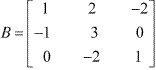∴ |B| = 1 × 3 - 2× (-1) - 2(2)
= 3 + 2 - 4
= 5 - 4 = 1
Now, A11 = 3, A12 = 1, A13 = 2
A21 = 2, A22 = 1, A23 = 2
A31 = 6, A32 = 2, A33 = 5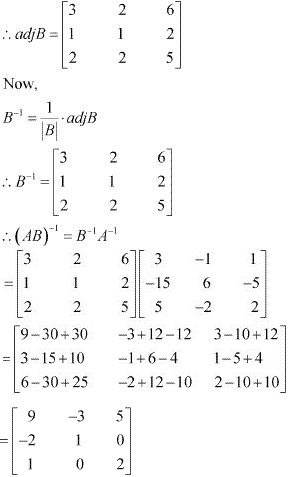8. Let A =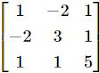verify that
(ii) (A-1)-1 = A
Solution
A =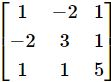∴ |A| = 1 (15 - 1) + 2(-10 - 1) + 1(-2 - 3) = 14 - 22 - 5= -13
Now,  A11 = 14, A12 = 11, A13 = -5
A21 = 11, A22 = 4, A23 = -3
A31 = -5, A32 = -3, A13 = -1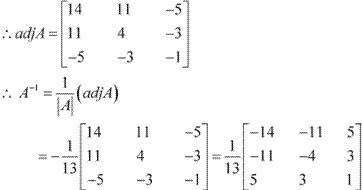(i) |adj A| = 14(-4 - 9) - 11(-11 - 15) - 5(-33 + 20)
= 14(-13) - 11(-26) - 5(-13)
= -182 + 286 + 65 = 169
we have ,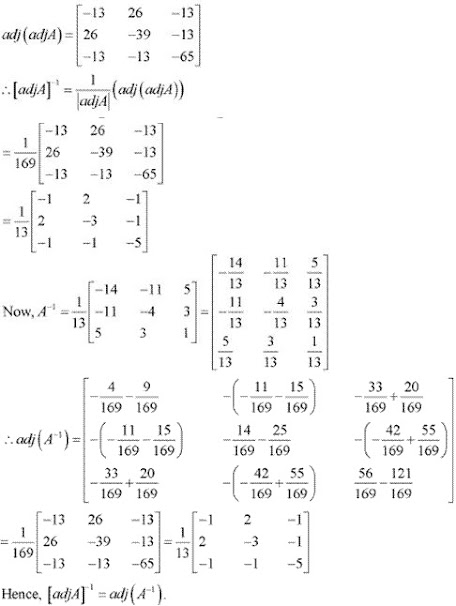(ii) We have show that :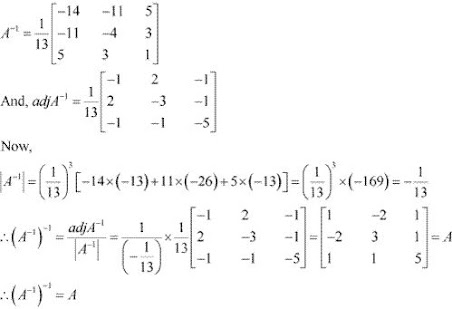9. Evaluate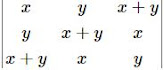Solution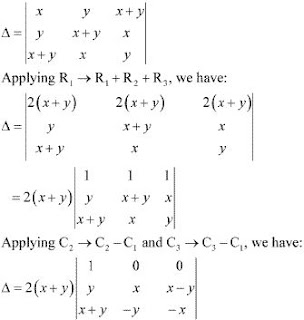10. Evaluate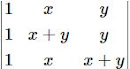Solution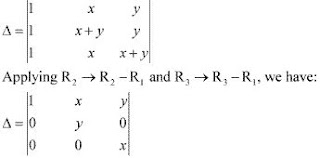Expanding along C1 , we have :
Δ = 1(xy - 0) = xy

11. Using properties of determinants, prove that :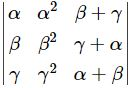= (β - γ) (γ - α)(α - β) (α + β + γ)
Solution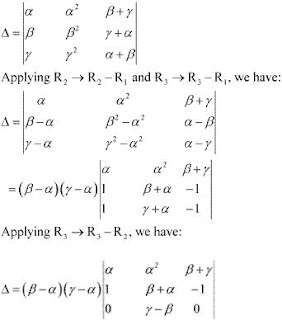Expanding along R3 , we have :
Δ =  (β - α) (γ - α)[- (γ - β)( - α - β - γ)]
= (β - α) (γ - α) (γ - β)(α + β + γ)
= (α - β) (β - γ) (γ - α)(α + β + γ)
Hence, the given result is proved.

12. Using properties of determinants, prove that :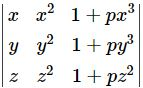= (1 + pxyz)(x - y)(y - z)(z -x), where p is any scalar.
Solution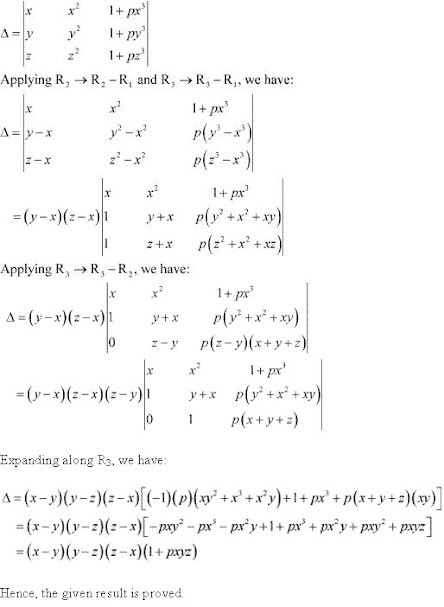13. Using properties of determinants, prove that :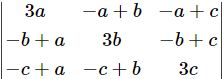= 3(a + b + c)(ab + bc + ca)
Solution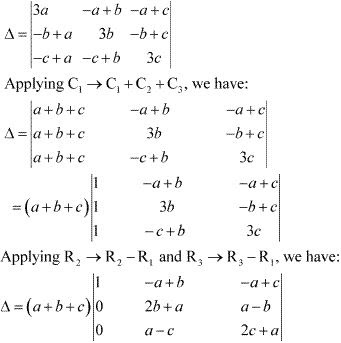Expanding along C1 , we have :
Δ = (a + b + c) [(2b + a)(2c + a) - (a - b)(a - c)]
= (a + b +c)[4abc + 2ab + 2ac + a2 - a2 + ac + ba - bc]
= (a + b + c)(3ab + 3bc + 3ac)
= 3(a + b + c)(ab + bc + ca)
Hence, the given result is proved.

14. Using properties of determinants, prove that :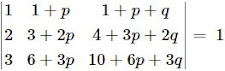Solution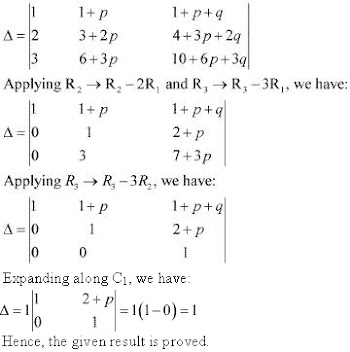15 . Solve the system of the following equations
2/x + 3/y + 10/z = 4
4/x - 6/y + 5/z = 1
6/x + 9/y - 20/x = 2
Solution
Let 1/x = p, 1/y = q, 1/z = r.
Then the given system of equations is as follows :
2p + 3q + 10r = 4
4p - 6q + 5r = 1
6p + 9q - 20r = 2
This system can be written in the form of AX = B, where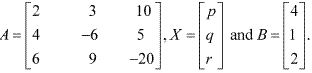Now,
|A| = 2(120 - 45) - 3 (-80 - 30) + 10(36 + 36)
= 150 + 330 + 720
=1200
Thus, A is non - singular. Therefore, its inverse exists.
Now,
A11 = 75, A12 = 110, A13 = 72
A21 = 150, A22 = -100, A23 = 0
A31 = 75, A32 = 30, A33 = -24
∴ A-1 = 1/|A| [adj A]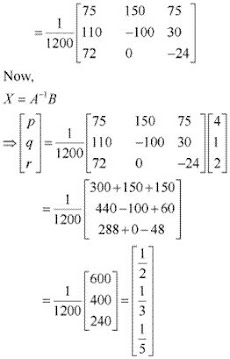∴ p = 1/2, q = 1/3, and r = 1/5
Hence, x = 2, y = 3, and z = 5.

If a, b, c, are in A.P., then the determinant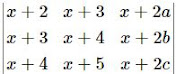A. 0
B. 1
C. x
D. 2x
Solution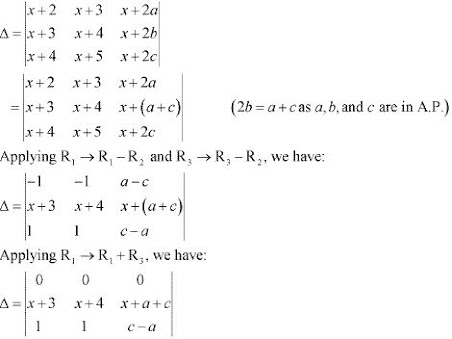Here, all the elements of the first row (R1 ) are zero.
Hence, we have Δ = 0.

18. If x, y, z are nonzero real numbers, then the inverse of matrix A =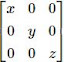is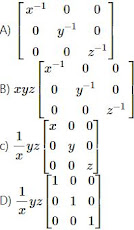Solution
A =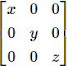∴ |A| = x(yz - 0) = xyz ≠ 0
Now,  A11 = yz, A12 = 0, A13 = 0
A21 = 0, A22 = xz, A23 = 0
A31 = 0, A32 = 0, A33 = xy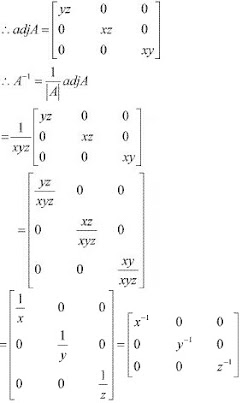19. Choose the correct answer .
Let A =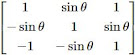where 0 ≤ θ ≤ 2π, then

A. Det (A) = 0
B. Det (A) ∈ (2, ∞)
C. Det (A) ∈ (2, 4)
D. Det (A)∈ [2, 4]

Solution

A =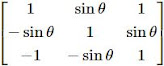∴ |A| = 1(1 + sin2 θ) - sinθ( - sinθ + sinθ) + 1 (sin2 θ + 1)
= 1 + sin2 θ + sin2 θ + 1
= 2 + 2sin2 θ
= 2(1 + sin2 θ)
Now, 0 ≤ θ ≤ 2π
⇒ 0 ≤ sinθ  ≤ 1
⇒ 0 ≤ sin2 θ  ≤ 1
⇒ 1 ≤ 1 + sin2 θ  ≤ 2
⇒ 2 ≤ 2(1 + sin2 θ)  ≤ 2
∴ Det (A) ∊ [2, 4]